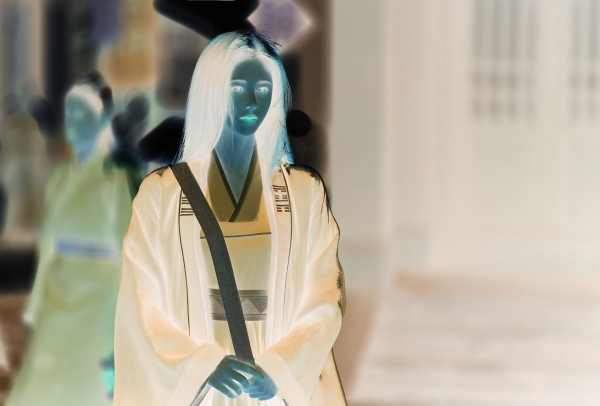WHCSRL 技术网

# Python 计算机视觉（四）—— OpenCV进行图像算数与逻辑运算

1. 图像加法运算

（1）随机生成灰度图像

（2）读取原始图像

（3）两图像相加

（4）图像像素点加常数

2. 图像减法运算

（1）减小亮度

（2）还原添加灰度图像的图像

3. 图像与运算

4. 图像或运算

5. 图像异或运算

6. 图像非运算

7. 图像逻辑运算的意义

## （1）随机生成灰度图像

``import numpy as npimport cv2#np.random.randint(a, b)生成大于等于 a 小于 b 的随机整数img_gray = np.random.randint(0, 256, size=[406, 600], dtype=np.uint8)#生成一张 406*600 的随机灰度图cv2.namedWindow("gray")cv2.imshow("gray", img_gray)cv2.waitKey(delay = 0)``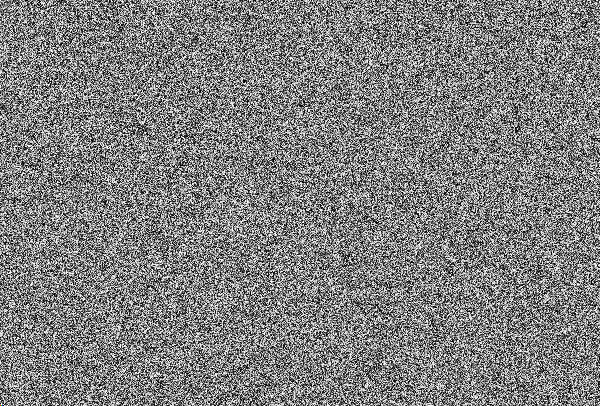## （2）读取原始图像

``import numpy as npimport cv2 img1 = cv2.imread('E:From ZhihuFor the deskde.jpg') #读取图像cv2.namedWindow("awindow")    #创建窗口img1_1 = cv2.resize(img1, dsize= None, fx = 0.5, fy = 0.5)#对图像尺寸进行调整cv2.imshow("awindow", img1_1)   #显示图像cv2.waitKey(delay = 0)    #等待print(img1_1.shape)   #读取图像信息``(406, 600, 3)  图像的分辨率以及通道，之前的灰度图像就是以这个图像的大小生成的

## （3）两图像相加

``"""Author:Huijundate:2021/10/15"""import numpy as npimport cv2 img1 = cv2.imread('E:From ZhihuFor the deskde.jpg')  #读取图像cv2.namedWindow("awindow") #创建窗口img1_1 = cv2.resize(img1, dsize= None, fx = 0.5, fy = 0.5)#调整大小img_gray0 = cv2.cvtColor(img1_1, cv2.COLOR_BGR2GRAY)          #对图像进行灰度处理cv2.imshow("awindow", img_gray0)   #显示图像cv2.waitKey(delay = 0) #等待 img_gray = np.random.randint(0, 256, size=[406, 600], dtype=np.uint8)#生成随机灰度图cv2.namedWindow("gray") cv2.imshow("gray", img_gray)print(img_gray.shape) #读取灰度图属性cv2.waitKey(delay = 0) final_img = cv2.addWeighted(img_gray0, 0.8, img_gray, 0.2, 8) #将两个图相加cv2.namedWindow("windowfinal")cv2.imshow("windowfinal", final_img)cv2.waitKey(delay = 0)````````final_img = cv2.addWeighted(img_gray0, 0.5, img_gray, 0.5, 8)
``````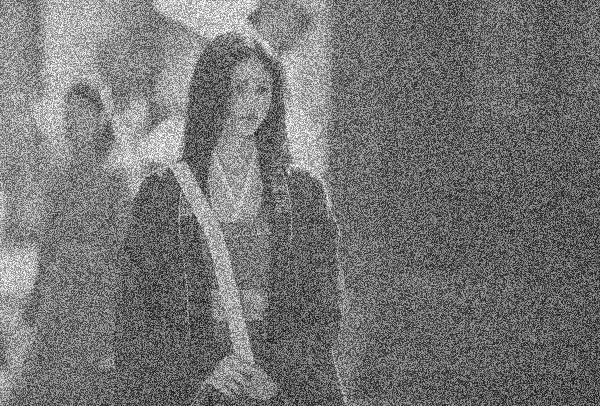## （4）图像像素点加常数

``Mix = np.ones(img1_1.shape, dtype=np.uint8)*50 #生成一个单位矩阵并乘以50，单位矩阵维度和 img1_1 相同Addimg = cv2.add(Mix, img1_1)   #给每一个像素点加50cv2.namedWindow("Addimg")cv2.imshow("Addimg", Addimg)cv2.waitKey(delay = 0)``# 2. 图像减法运算

## （1）减小亮度

`cv2.subtract() 函数可以实现图像之间的减法`

``img1_2 = cv2.subtract(Addimg, Mix)  #在得到的图像中减去矩阵cv2.namedWindow("subimg")cv2.imshow("subimg", img1_2)cv2.waitKey(delay = 0)``

得到的效果如下：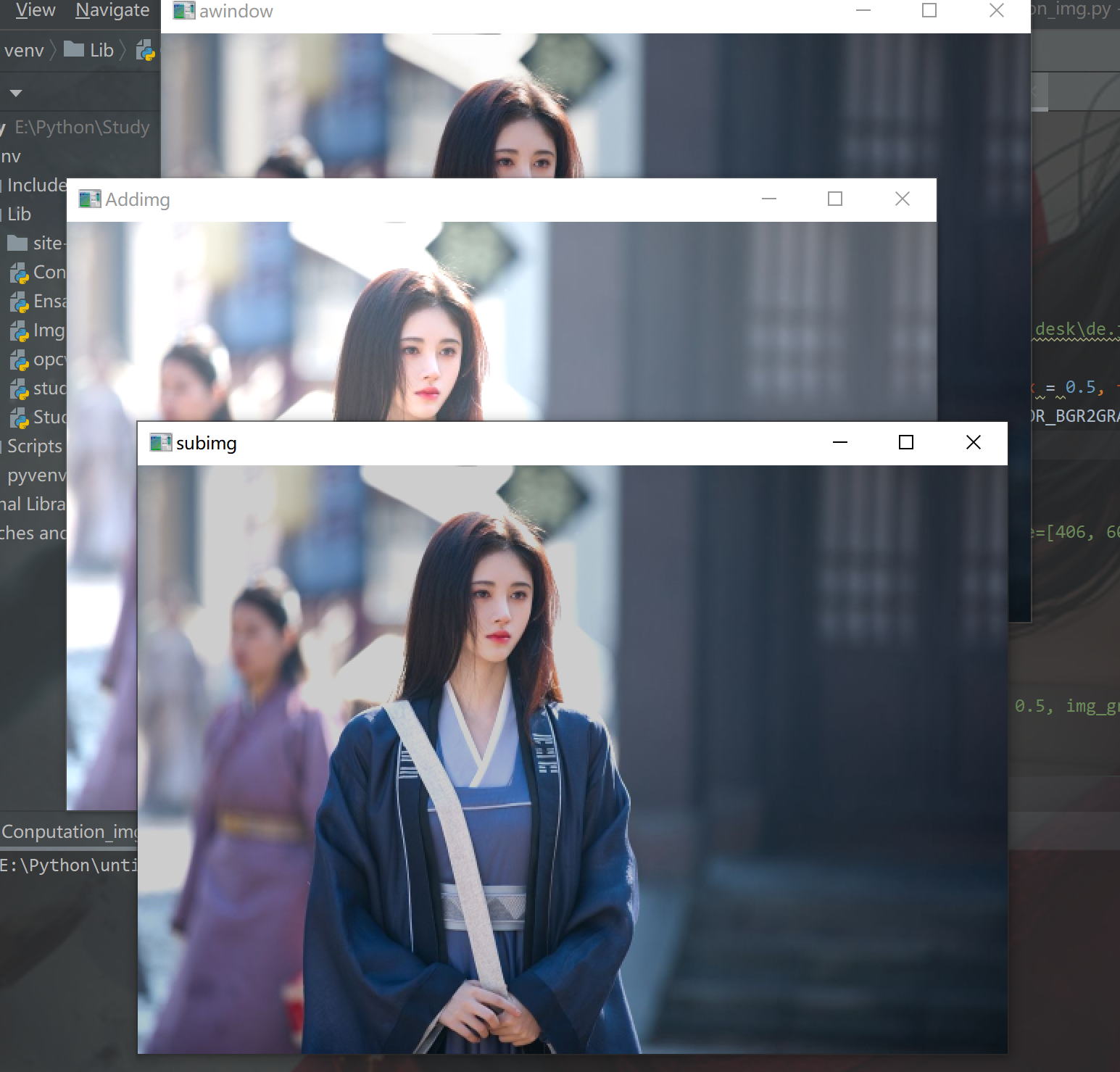## （2）还原添加灰度图像的图像

``img1_3 = cv2.subtract(final_img, img_gray)cv2.namedWindow("subimg1")cv2.imshow("subimg1", img1_3)cv2.waitKey(delay = 0)``可以看出减去灰度图像得到的图像并没有将原图复原出来，这是因为我们之前将两图像进行相加是按照权值相加的，所以直接减去灰度图像会使整个图像亮度降低

# 3. 图像与运算

``img1_4 = cv2.bitwise_and(img_gray0, img_gray)  #对两种图像进行与操作cv2.namedWindow("And")cv2.imshow("And", img1_4)cv2.waitKey(delay = 0)``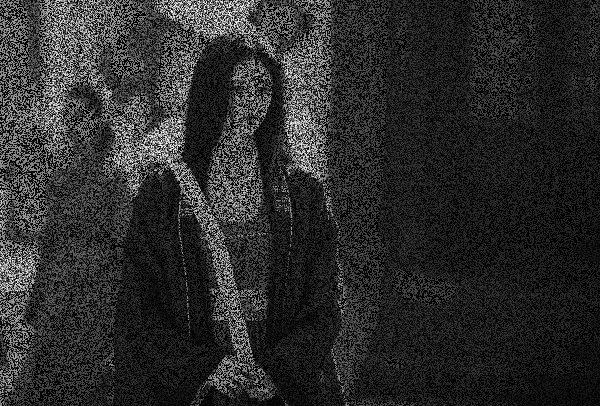``"""Author:Huijundate:2021/10/15"""import numpy as npimport cv2 img1 = cv2.imread('E:From ZhihuFor the deskde.jpg')cv2.namedWindow("beauty0")img1_1 = cv2.resize(img1, dsize= None, fx = 0.5, fy = 0.5)cv2.imshow("beauty0", img1_1)print(img1_1.shape)cv2.waitKey(delay = 0) img1_5 = cv2.imread('E:From ZhihuFor the deskde1.jpg') img1_50 = cv2.resize(img1_5, dsize= None, fx = 0.834, fy = 0.903) #调整大小和前一幅图像一致cv2.namedWindow("beauty1")cv2.imshow("beauty1", img1_50)cv2.waitKey(delay = 0)print(img1_50.shape) img1_51 = cv2.bitwise_and(img1_1, img1_50)cv2.namedWindow("Add1")cv2.imshow("Add1", img1_51)cv2.waitKey(delay = 0)``

得到的图像：。。。。。离了个大谱！beauty0 和 beauty1 都没了

# 4. 图像或运算

``````img1_51 = cv2.bitwise_or(img1_1, img1_50) #对两图像进行或运算
``````# 5. 图像异或运算

``````img1_51 = cv2.bitwise_xor(img1_1, img1_50) #对两图像进行异或操作
``````# 6. 图像非运算

``img1_51 = cv2.bitwise_not(img1_1) #对读取的图像进行非操作cv2.namedWindow("Not")cv2.imshow("Not", img1_51)cv2.waitKey(delay = 0)``